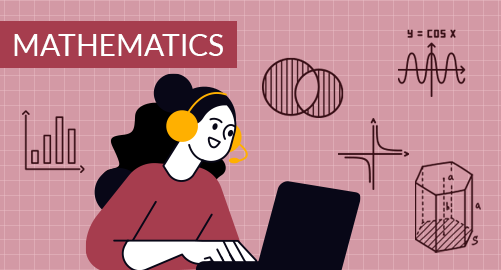#### Need Help?

Get in touch with usMathematics

Grade 5 Mathematics in the USA provides students with foundational mathematical skills and concepts to prepare students for more advanced mathematical challenges. It covers various key areas, including numbers, model strategies to find arithmetical operations, fractions, decimals, introduction to algebra, geometry, measurements, area and volume, and data handling Students develop a deeper understanding of mathematical principles and problem-solving techniques, enhancing their ability to apply mathematics in real-life scenarios.

## What you'll learn

• 1

Number System - Numbers, Place Value, Rounding Off, Basic Number Operations including addition, subtraction, Multiplication and division

• 2

Fractions and Decimals -Multiplication of fractions, model strategies to multiply fractions, division of fractions, division of whole numbers, division of mixed numerals, basic arithmetic operations on decimals, and word problems.

• 3

Algebra- Number sequence and patterns, solving algebraic expressions and numerical expressions, use of rules and patterns.

• 4

Geometry- Analyzing 2D shapes such as triangles, and quadrilaterals.

• 5

Measurements - Volume of 3D shapes, converting measurements such as length, mass, capacity, and weight.

• 6

Data Handling - Organizing the data, making line plots, and analyzing line plots.

• 7

Time - Units of time, reading time, calendars, timetables, time patterns

• 8

Graphs and plane - concepts of coordinate plane and regions, locating graph points, solving using ordered pair, and analyzing the graph.

## Skills you'll learnNumeracy Skills - Understanding numbers and their place Values, mastering essential arithmetic operations, and Estimating Results.Arithmetic Operations - Multiplication and division on fractions and mixed numerals, arithmetic operations on decimals, Real-world Problem Solving, Rounding Off, and Computational Fluency.Algebraic Thinking - Understanding algebraic expressions and equations, Recognizing, and applying the sequences, and creating rules for the patterns.Geometrical Skills and Spatial Relationships -A deeper understanding of 2D shapes, classification of triangles and quadrilaterals, and recognizing shapes in real life.Measurement skills - Calculating the distance, Finding the Space Occupied, understanding cardinal directions, Conversions of units, and finding the volume of prism.Data Analysis and Predictions - Data Organization, create graphs, interpret data, Calculate Predictions.Reasoning- Plan and schedule activities, Estimate the time, Understanding the passage of Timesketching and analyzing - understanding the coordinate plane, and regions of the plane, identifying the location of the points, sketching the graphs, and analyzing of graph.